Modeling charge polarization voltage for large lithium-ion batteries in electric vehiclesModeling charge polarization voltage for large lithium-ion batteries
in electric vehicles

Yan Jiang, Caiping Zhang, Weige Zhang, Wei Shi, Qiujiang Liu

School of Electrical Engineering, Beijing Jiaotong University (China)

Accepted: June 2013

Jiang, Y., Zhang, C., Zhang, W., Shi, W., & Liu, Q. (2013). Modeling charge polarization voltage for large lithium-ion batteries in electric vehicles. Journal of Industrial Engineering and Management, 6(2), 686-697. http://dx.doi.org/10.3926/jiem.895

---------------------

Abstract:

Purpose: Polarization voltage of the lithium-ion battery is an important parameter that has direct influence on battery performance. The paper aims to analyze the impedance characteristics of the lithium-ion battery based on EIS data.

Design/methodology/approach: The effects of currents, initial SOC of the battery on charge polarization voltage are investigated, which is approximately linear function of charge current. The change of charge polarization voltage is also analyzed with the gradient analytical method in the SOC domain. The charge polarization model with two RC networks is presented, and parts of model parameters like Ohmic resistance and charge transfer impedance are estimated by both EIS method and battery constant current testing method.

Findings: This paper reveals that the Ohmic resistance accounts for much contribution to battery total polarization compared to charge transfer impedance.

Practical implications: Experimental results demonstrate the efficacy of the model with the proposed identification method, which provides the foundation for battery charging optimization.

Originality/value: The paper analyzed the impedance characteristics of the lithium-ion battery based on EIS data, presented a charge polarization model with two RC networks, and estimated parameters like Ohmic resistance and charge transfer impedance.

Key words: charge polarization voltage, modeling, lithium-ion batteries, electric vehicles

---------------------

1. Introduction

Lithium-ion batteries have been the subject of significant research and development in recent years and become a promising alternative for power units in hybrid electric vehicles (HEV) and electric vehicles (EV) due to their high nominal voltage, high energy density, long life and none memory effect. As one of power supplies, the performance of lithium-ion batteries will have direct effects on the driving performance and operating economy of the vehicle. It was reported that battery charging contributed more to capacity fading compared to battery discharging (Dubarry, Svoboda, Hwu, & Liaw, 2007a, 2007b). It is therefore necessary to investigate the charging characteristics and associated affect factors of the battery.

The lithium-ions migrate from positive to negative electrode during charging, embedding in porous electrode material in a process known as intercalation (Linden & Reddy, 2002). The polarization voltage representing the change of cell voltage from its open-circuit voltage will appear during the charge and discharge state. From electrochemistry point of view, Ohzuku Yamato, Kawai and Ariyoshi (2008) reported the steady-state polarization measuring method of a lithium-ion cell and demonstrated its efficacy (Ohzuku et al., 2008). Nakayama, Iizuka, Shiiba et al., (2011) reported the electrochemical model with a two-phase system of LiFeO4 and FePO4to describe the battery polarization profiles (Nakayama et al., 2011). Nyman, Zavalis, Elger Behm and Lindbergh (2010) reported the cell polarization profiles at various states of charge (SOC) and suggested the mass transport by diffusion mainly contributed to the total polarization (Nyman et al., 2010). The chemical parameters and detailed knowledge of the battery construction and material properties are however normally unavailable, which bring difficulty in estimating polarization voltage of the battery in practical application. In this respect, the present study aims to investigate the characteristics of charge polarization voltage for large lithium-ion battery based on both electrochemistry impedance spectroscopy (EIS) measurement data and battery charge testing results, analyze the effects of initial SOC and charge current on charge polarization voltage and their relationships, and establish the charge polarization voltage simulation model as the basis of battery charging optimization.

2. Battery charge polarization voltage characterization

2.1. Experimental details

The sample cell used in this study is LiMn2O4 battery with the nominal capacity of 90 Ah. The test bench of the EIS and battery external performance are shown in Figure 1(a) and Figure 1(b), respectively. The external electronic load EL300with one-quadrant potentiostats shown in Figure 1(a) was used to satisfy the current requirement since the internal resistance of the sample battery was tiny and the high current was needed to get required incremental voltage value. The EL300potentiostats has the maximum current of 100A, which can be operated in both potentiostatic and galvanostatic modes.

The Arbin battery testing system was used to carry out the battery charge and discharge test. The testing system is capable of charging and discharging the battery at a maximum rate of 200A. The battery was placed in the temperature thermostat to be kept at a specified temperature environment during test.

 (a)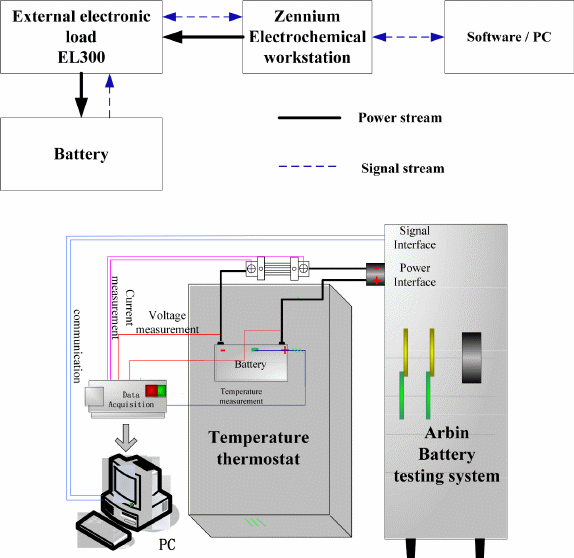(b)

Figure 1. (a) EIS test bench for large lithium-ion cell; (b) Schematic diagram of battery testing system

2.2. Characteristic analysis of EIS

Electrochemical impedance spectroscopy (EIS) is a measuring method in frequency domain. It investigates the electrode system by measuring impedance spectroscopy in a wide range of frequency, which can get more information about dynamic and electrode interface structure than other conventional electrochemical methods (Nyman et al., 2010; Shi, 2001; Cao & Zhang, 2002). When the equivalent circuit model is given a sinusoidal input current signal on a certain angular frequency, the external of the measuring circuit will get a voltage signal of the same angular frequency; we call the frequency response function of the circuit model “equivalent circuit impedance”. Cells’ electrode reaction is quite complicated, when a sinusoidal perturbation input is flowing in the cell system, change response conditions of dynamic factors such as the electrode potential and the concentration of substance near the electric double layer will affect the impedance spectrum analysis. It is found that when the electrode response and disturbance are approximately linear with the linear conditions, it can be described using the equivalent circuit model in the corresponding frequency range.

To study the battery electrode dynamics, impedance spectroscopy technology is used to measure the frequency response from 0.1Hz to 10 kHz of a LiMn2O4 battery. It is suggested that a capacitive reactance arc similar to the electric double layer capacitor is existed in impedance spectroscopy analysis; the information is therefore obtained about the change of electrode reaction interface and electrode interface dynamics.

By testing the impedance spectroscopy of LiMn2O4battery at various SOC, impedance spectrograms are gotten on the complex plane of different points of 3%, 10%, 20%, 30%, 40%, 50% and 60%as shown in Figure 2. Each point is measured three times at room temperature to decrease the testing error since the impedance of the 90Ah battery is very small. The battery electrode reaction rate is higher, it performs as a capacitive reactance arc consisted of electric double layer capacitor and polarization resistance in parallel from 2Hz to 300Hz, while at lower frequency the diffusion impedance spectroscopy which presents the diffusion process is not obvious. This suggests that new batteries’ impedance characteristic is mainly determined by electrochemical polarization resistance Rpa and battery Ohmic internal resistance RΩ.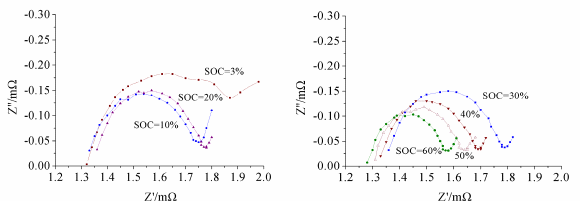Figure 2. EIS of LiMn2O4 battery at various SOC

The fitting results of the RC first-order circuit model are shown in Table 1. The Rpa gotten by fitting in this frequency range mainly contains electrochemical polarization resistance resulted from electrode reaction rate, therefore, the fitting result contributes to analyze establishment and the regression rate of battery polarization accurately. The RpaQY signifies the time constant of charge transfer polarization, which reflects the polarization reaction rate of the battery at this SOC point.

Figure 3 shows the relationship between Rpa/RpaQY and different SOC points. When the SOC is at the low side, Rpa is higher. By experiment we find that in the range of 0%-10% the polarization voltage produced at the beginning of the charge is higher, in the range of 10%‑30% the polarization voltage produced is stable, in the range of 40%-60% the Rpa decreases gradually, and the polarization reaction rate is increased, which means in this range the electrochemical polarization degree is lower.

 SOC/% 3 10 20 30 40 50 60 RΩ/mΩ 1.32 1.33 1.35 1.36 1.33 1.31 1.29 QY 43.38 34.39 26.97 26.19 28.62 29.35 29.95 Qn 0.76 0.81 0.84 0.85 0.86 0.88 0.9 Rpa/mΩ 0.59 0.41 0.4 0.41 0.34 0.3 0.26 RpaQY 25.5 14.2 10.8 10.8 9.8 8.9 7.9

Table 1. EIS test data based model parameters as a function of SOC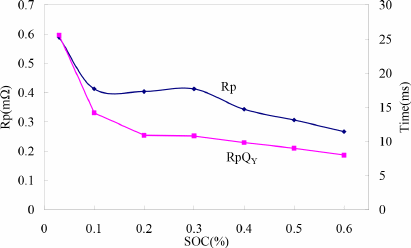Figure 3. Relation between Rp\RpQY and different SOC points

2.3. Effects on charge polarization voltage

State of charge

When connected to a charger, the terminal voltage of the battery can be expressed as:(1)

Where VOC represents open-circuit cell voltage, IRO denotes Ohmic loss of the battery concerned with contact resistance, electrolyte resistance, I expresses battery charge current, Vp describes the polarization voltage in respect of mass transport or concentration polarization and charge transfer or activation polarization of the battery. The open-circuit voltage can be obtained by measuring the terminal voltage after the battery left in an open circuit condition for a long time when got to steady state. The Ohmic resistance Ro could be got through battery voltage response data at pulse current. And the polarization voltage can be calculated based on Equation (1).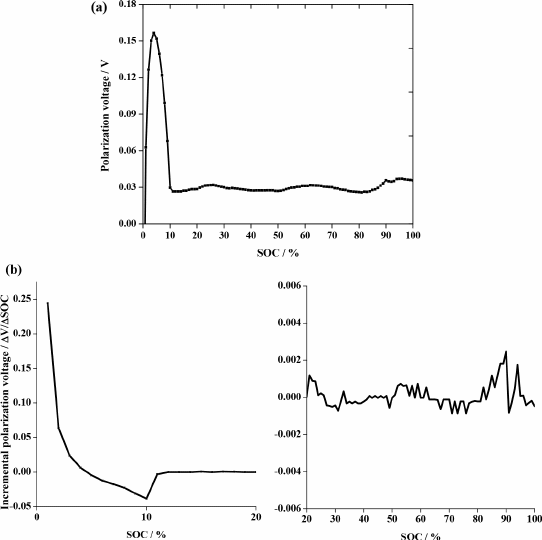Figure
4. (a) Battery charge polarization voltage variation with SOC; (b) Incremental
charge polarization voltage as a function of SOC

Figure 4(a) and (b) reports the charge polarization voltage and incremental charge polarization voltage changing with SOC. In this experiment, the battery was first charged with a constant current of C/3 until the battery voltage reached the maximum charge voltage, then was charged at maximum voltage till the current dropped to 5A. From the data in Figure 4, it is seen that the polarization voltage is dramatically increasing till the battery SOC arrives around 5%, and then drops fast into a relatively steady state after about 10 % nominal capacity of the battery was recharged, which the incremental polarization voltage appears “valley” shown in Figure 4(b). The polarization voltage of SOC ranging from 0% to 10% is much higher that of after 10% SOC, which is also demonstrated by EIS results shown in Table 1. From 10% to 80% SOC, the polarization voltage fluctuates within 10 mV. The polarization voltage is gradually going up between 80% and 90% SOC, suggesting that the polarization of the battery at this period is raising, and then maintains high level and does not increase till fully charged since the battery was charged at constant voltage and the current was gradually decreased. It is inferred that two fields which are from 0% to 10% SOC and from 80% to 100% SOC need to be charged at low current and not suitable for fast charging.

Figure 5(a) and (b) illustrates the battery charge polarization voltage and its initial polarization level at various initial SOC. It is found that the charge polarization voltage response characteristics of SOC ranging from 10% to 70% is similar to over damping dynamic system that the voltage starts fast increasing and then maintains nearly constant, which is much different from that of 0% SOC shown in Figure 4(a). In addition, the steady value of polarization voltage for various initial SOC differs from each other, especially for initial SOC of 10% and 60%; the difference arrives around 10mV. It is indicated that the charge polarization is affected by battery initial SOC. The effects of SOC on charge polarization voltage therefore need to be considered in modeling.Figure 5. (a) Charge polarization voltage characteristics at various initial SOC; (b) Initial polarization level vs. initial SOC (the battery was charged at the rate of C/3)

Charge current

The polarization voltage versus SOC profile at different charge current is reported in Figure 6. It is apparent that the polarization voltage increases with charge current increasing as expected. To further investigate the relationship of the polarization voltage and charge current of the battery, the data in Figure 6 was extracted at a specified SOC point, and then the polarization voltage would be obtained at different currents, which is illustrated in Figure 7. From Figure 7, it is shown that the polarization voltage is an approximately linear function of charge current, and the values are also related with battery SOC as described in detail in previous section.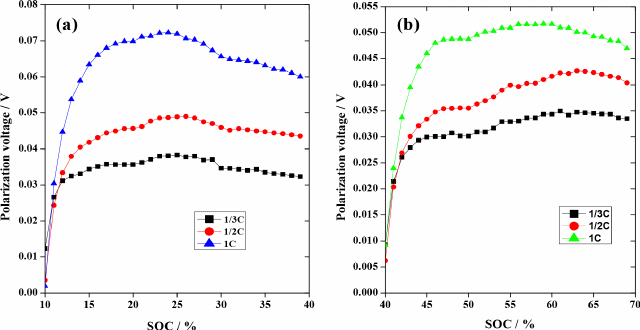Figure
6. Polarization voltage vs. SOC profile at different charge currents. (a) initial SOC = 10%;
(b) initial SOC = 40%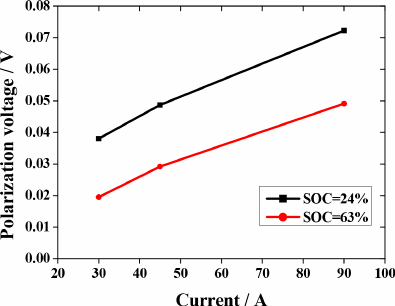Figure 7. Polarization voltage vs. charge current profile

3. Modeling and simulation

3.1. Model formulation

The equivalent circuit model, the neural network model and the simplified electrochemical model are most widely used in battery simulation. The equivalent circuit model based on the battery working principle describes the performance characteristics of the battery with circuit network, and it is suitable for many types of batteries. The RC network model is the most representative. From the above characteristic analysis, the establishment of the polarization voltagewith exponential function change rule is consistent with the structure characteristics of the circuit model. Polarization voltage consists of concentration polarization voltage and electrochemical polarization voltage. So it is reasonable to use the second order RC model to simulate the two parts of polarization effects respectively. The equivalent circuit model is shown in Figure 8.Figure 8. Equivalent circuit model of the battery

Based on Kirchhoff’s Law, the model can be described by the following equation: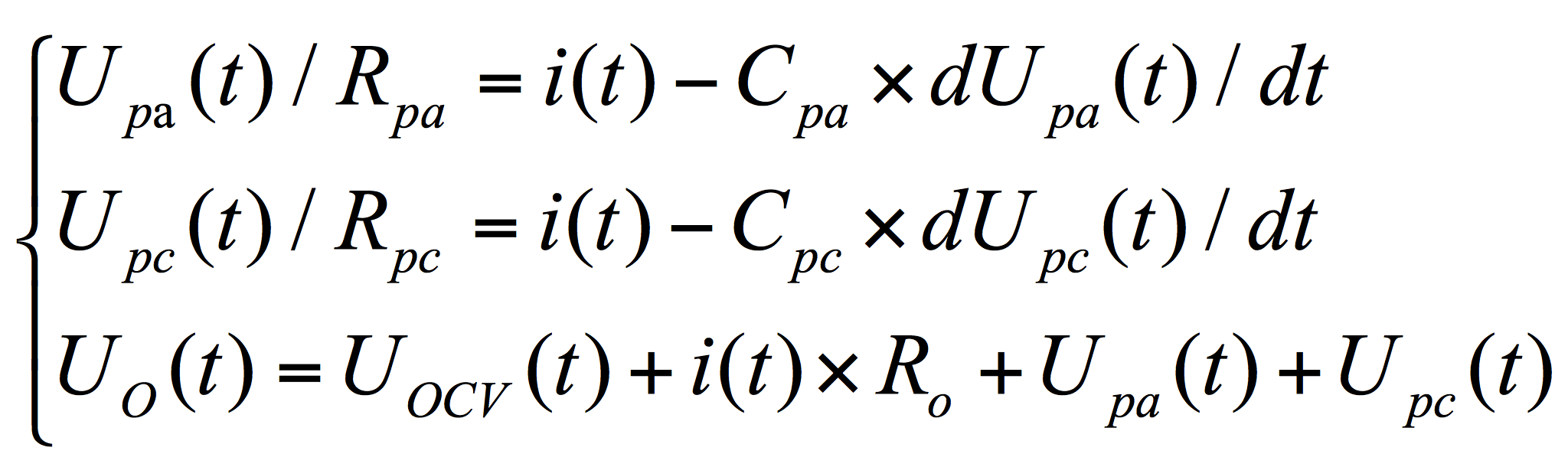(2)

And the solution of Equation (3) is given by: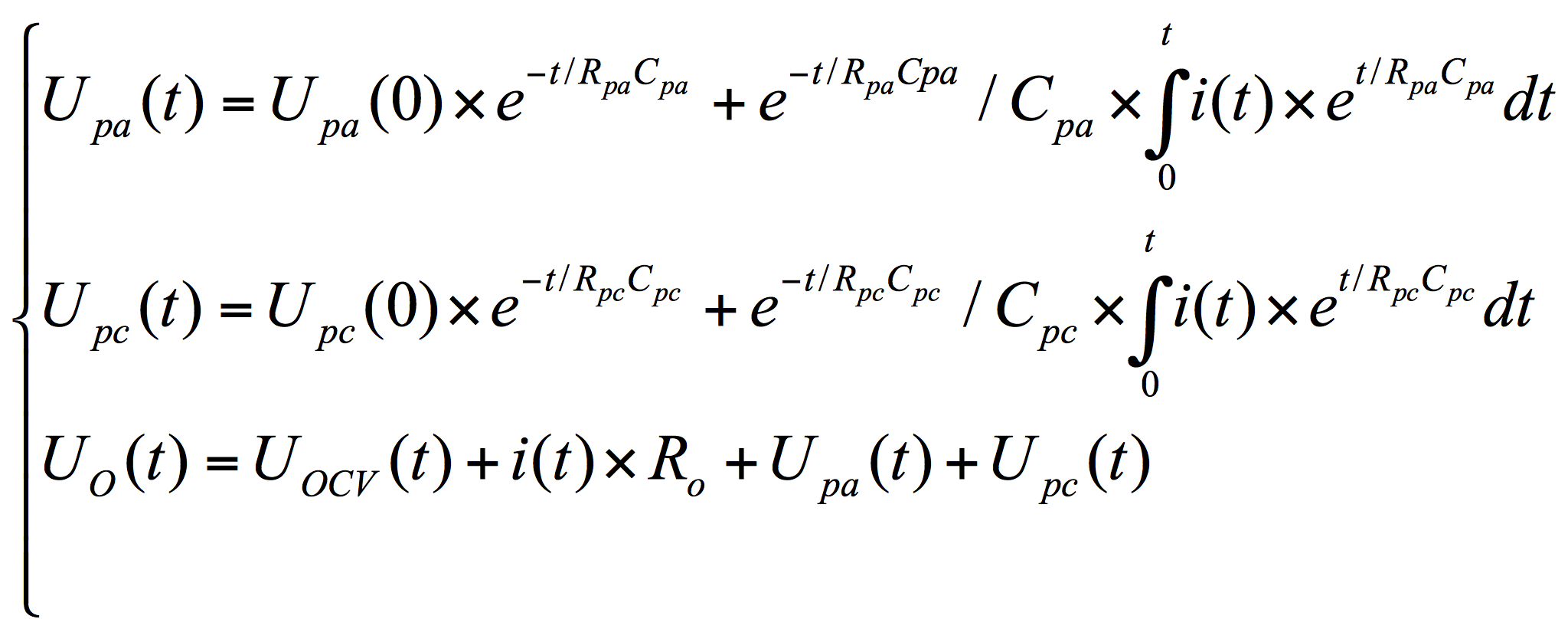(3)

The initial polarization voltage Upa (0), Upc (0) is equal to 0 after the battery was kept in an open circuit condition for a long time. The charge current I can be regarded as constant with time interval, and Equation (3) can be further simplified. The polarization voltage Up is the sum of Upa, Upc. The simplified equation can be expressed by:(4)

3.2. Identification of parameters

The model parameters of Ro, Rpa, Rpc, Cpa, Cpc need to be identified to simulate the charge polarization voltage dynamics. The Ohmic resistance Ro is significantly influenced by battery temperature. Ro can be regarded as constant at a specified temperature during the charging and discharging process. The dc internal resistance can be achieved by measuring battery terminal voltage response at a certain charge current since it performs pure resistance behavior. As for the Rpa, Rpc, Cpa, Cpc identificationssome rules can be found from the Up formula, in which the constant part equals the sum of the coefficients of two exponential functions. The constant is divided into two partsone is high frequency components expressing charge transfer polarization, the other is low frequency components describing mass transport polarization. According to time constant τ = RC, the parameters of Rpa, Rpc, Cpa, Cpc can be identified based on nonlinear least square method. The model parameters are listed in Table 2.

 SOC/% 10 20 30 40 50 60 70 Ro/mΩ 1.38 1.41 1.41 1.38 1.36 1.38 1.41 Rpa/mΩ 0.31 0.31 0.29 0.31 0.37 0.35 0.31 pa/s 25.19 76.74 122.23 140.28 84.02 158.29 139.59 Rpc/mΩ 0.72 0.72 0.68 0.72 0.88 0.83 0.72 pc/s 255.8 150.1 122.2 140.3 268.6 158.2 139.5

Table 2. Constant current test data based model parameters as a function of SOC

Compared to the data in Table 1, we can find that the values of parameter Rpa expressing battery activation polarization estimated by EIS method and constant current method are nearly the same, and both of them are around 0.3mΩ, which demonstrates the efficacy and accuracy of both methods. It is concluded that large lithium-ion battery can also be characterized by EIS test with high current. It is noticeable that the proposed model can get the estimation of parameter Rpc describing concentration polarization of the battery, nevertheless, EIS test could not illustrate concentration polarization characteristics in this experiment because of the frequency limit.

3.3. Validation

The Simulink model was established for simulating battery charge polarization. The initial SOC was set to 20%, and the parameters were varying with SOC increase during the simulation. The simulated and experimental polarization voltage is illustrated in Figure 9(a), and the estimate error is shown in Figure 9(b), respectively. It is found that the proposed model with identified parameters can effectively simulate the charge polarization dynamics of the battery, and the maximum estimation error is controlled within 3.5mV, satisfying the accuracy requirement of the battery usage in electric vehicles.Figure 9. (a) The simulated and measured polarization voltage of the battery for constant charging current; (b) The simulation error for the battery charge polarization model

4. Conclusions

The paper analyzes the impedance characteristics of the lithium-ion battery based on EIS data, revealing that the Ohmic resistance accounts for main contributions to battery total polarization compared to charge transfer impedance. The charge polarization voltage of the battery is involved with both initial SOC and operation current, and is approximately linear function of charge current. The charge polarization model with two RC networks is presented, and parts of model parameters are estimated by both EIS method and battery constant current testing method, which has nearly the same results. Experimental results demonstrate the efficacy of the charge polarization model with the proposed identification method, which provides the foundation for battery charging optimization.

References

Cao, C.N., & Zhang, J.Q. (2002). An Introduction to Electrochemical Impedance Spectroscopy. Beijing: Science Press.

Dubarry, M., Svoboda, V., Hwu, R., & Liaw, B.Y. (2007a). Capacity and power fading mechanism identiﬁcation from a commercial cell evaluation. Journal of Power Sources, 165, 566-572. http://dx.doi.org/10.1016/j.jpowsour.2006.10.046

Dubarry, M., Svoboda, V., Hwu, R., & Liaw, B.Y. (2007b). Capacity loss in rechargeable lithium cells during cycle life testing: The importance of determining state-of-charge. Journal of Power Sources, 174, 1121-1125. http://dx.doi.org/10.1016/j.jpowsour.2007.06.185

Linden, D., & Reddy, T.B. (Ed) (2002). Handbook of Batteries (3rd Edition). New York: McGraw‑Hill, chapter 35.

Nakayama, M., Iizuka, K., Shiiba, H., et al. (2011). Asymmetry in anodic and cathodic polarization proﬁle forLiFePO4 positive electrode in rechargeable Li ion battery. Journal of the Ceramic Society of Japan, 119(9), 692-696. http://dx.doi.org/10.2109/jcersj2.119.692

Nyman, A., Zavalis, T.G., Elger, R. Behm, M., & Lindbergh, G. (2010). Analysis of the Polarization in a Li-Ion Battery Cell by Numerical Simulations. Journal of The Electrochemical Society, 157(11), A1236-A1246. http://dx.doi.org/10.1149/1.3486161

Ohzuku, T., Yamato, R., Kawai, T., Ariyoshi, K. (2008). Steady-state polarization measurements of lithium insertion electrodes for high-power lithium-ion batteries. Journal of Solid State Electrochemistry, 12, 979-985. http://dx.doi.org/10.1007/s10008-007-0464-4

Shi, M.L. (2001). Impedance Spectroscopy Principles and Applications. Beijing: National Defense Industry Press.